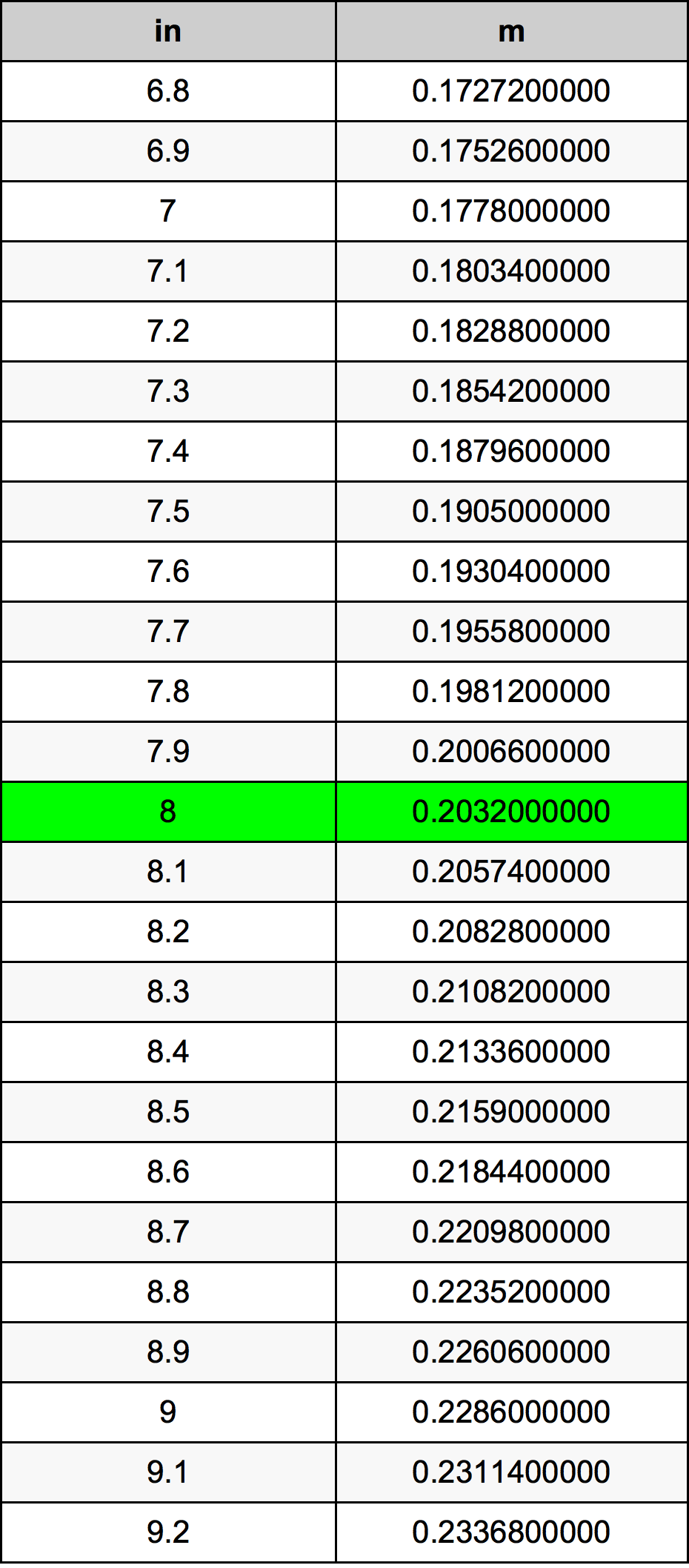Inches To Meters

# 8 in to m8 Inches to Meters

in
=
m

## How to convert 8 inches to meters?

 8 in * 0.0254 m = 0.2032 m 1 in
A common question is How many inch in 8 meter? And the answer is 314.960629921 in in 8 m. Likewise the question how many meter in 8 inch has the answer of 0.2032 m in 8 in.

## How much are 8 inches in meters?

8 inches equal 0.2032 meters (8in = 0.2032m). Converting 8 in to m is easy. Simply use our calculator above, or apply the formula to change the length 8 in to m.

## Convert 8 in to common lengths

UnitLength
Nanometer203200000.0 nm
Micrometer203200.0 µm
Millimeter203.2 mm
Centimeter20.32 cm
Inch8.0 in
Foot0.6666666667 ft
Yard0.2222222222 yd
Meter0.2032 m
Kilometer0.0002032 km
Mile0.0001262626 mi
Nautical mile0.0001097192 nmi

## What is 8 inches in m?

To convert 8 in to m multiply the length in inches by 0.0254. The 8 in in m formula is [m] = 8 * 0.0254. Thus, for 8 inches in meter we get 0.2032 m.

## 8 Inch Conversion Table## Alternative spelling

8 Inches to m, 8 Inches in m, 8 Inch to m, 8 Inch in m, 8 in to m, 8 in in m, 8 Inch to Meters, 8 Inch in Meters, 8 Inches to Meter, 8 Inches in Meter, 8 Inches to Meters, 8 Inches in Meters, 8 in to Meter, 8 in in Meter